# Previous year questions (2014-21): Motion in a Plane Notes | Study Physics Class 11 - NEET

## NEET: Previous year questions (2014-21): Motion in a Plane Notes | Study Physics Class 11 - NEET

The document Previous year questions (2014-21): Motion in a Plane Notes | Study Physics Class 11 - NEET is a part of the NEET Course Physics Class 11.
All you need of NEET at this link: NEET

Q 1. A car starts from rest and accelerates at 5 m/s2. At t = 4 s, a ball is dropped out of a window by a person sitting in the car. What is the velocity and acceleration of the ball at t = 6 s ?    
A: 20√2m/s, 0 m/s2
B: 20√2m/s, 10 m/s2
C: 20 m/s,5 m/s2
D: 20 m/s, 0
Ans:
B
Solutions:

velocity of car at t = 4 sec is
v = u + at  v = 0 + 5(4)
= 20 m/s
At t = 6 sec
acceleration is due to gravity
∴ a = g = 10 m/s
vx = 20 m/s (due to car)
vy = u + at
= 0 + g(2) (downward)
= 20 m/s (downward)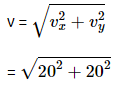= 20√2 m/s.

Q 2. A particle moving in a circle of radius R with a uniform speed takes a time T to complete one revolution. If this particle were projected with the same speed at an angle 'θ'to the horizontal, the maximum height attained by it equals 4R. The angle of projection,θ, is then given by:    
A: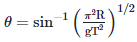B: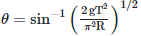C: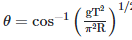D: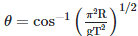Ans: B
Solutions: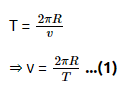Q 3. The speed of a swimmer in still water is 20 m/s. The speed of river water is 10 m/s and is flowing due east. If he is standing on the south bank and wishes to cross the river along the shortest path, the angle at which he should make his strokes w.r.t. north is given by:    
A: 30° west
B: 0° west
C: 60° west
D: 45° west
Ans:
A
Solution:
VSR = 20m/s
VRG =  10m/s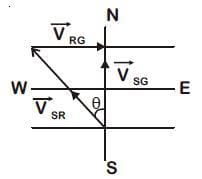sin θ = 10/20
sin θ = 1/2
θ = 300 west

Q 4. A particle moving with velocity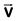is acted by three forces shown by the vector triangle PQR. The velocity of the particle will: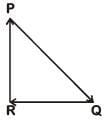A: Increase
B: Decrease
C: Remain constant
D: Change according to the smallest force
Ans:
C
Solution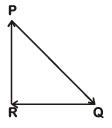As forces are forming closed loop in same order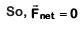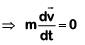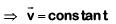Q 5. The x and y coordinates of the particle at any time are x = 5t – 2t2 and y = 10t respectively, where x and y are in meters and t in seconds. The acceleration of the particle at t = 2s is:-    
A: 5 m/s2
B: – 4 m/s2
C: – 8 m/s2
D: 0
Ans:
B
Solution: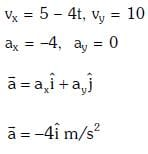Q 6. One end of string of length l is connected to a particle of mass 'm' and the other end is connected to a small peg on a smooth horizontal table. If the particle moves in circle with speed 'v' the net force on the particle (directed towards centre) will be (T represents the tension in the string):-    
A: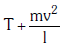B: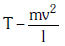C: Zero
D: T
Ans:
D
Solution: Net force on the particle in uniform circular motion is centripetal force, which is provided by the tension in string.

Q 7. If the magnitude of sum of two vectors is equal to the magnitude of difference of the two vectors, the angle between these vectors is:    
A: 180 deg
B: 0 deg
C: 90 deg
D: 45 deg
Ans:
C
Solution: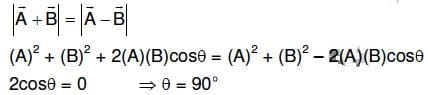Q 8. A ship A is moving Westwards with a speed of 10 km h-1 and a ship B 100 km South of A, is moving Northwards with a speed of 10 km h-1. The time after which the distance between them becomes shortest, is:    
A: 10√2h
B: 0 h
C: 5h
D: 5√2h
Ans:
C
Solution: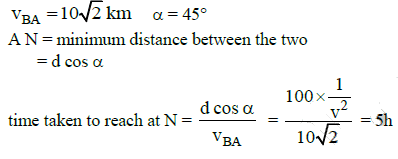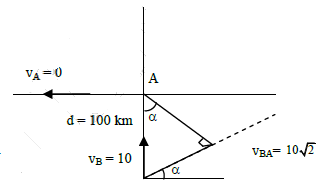Q 9. A particle is moving such that its position coordinates (x, y) are (2m, 3m) at time t = 0, (6m, 7m) at time t = 2s and (13m, 14m) at time t= 5s. Average velocity vector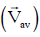from t = 0 to t = 5s is:    
A: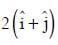B: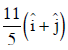C: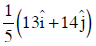D: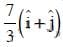Ans:
B
Solution:
Average velocity Vector,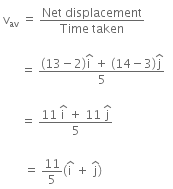The document Previous year questions (2014-21): Motion in a Plane Notes | Study Physics Class 11 - NEET is a part of the NEET Course Physics Class 11.
All you need of NEET at this link: NEETUse Code STAYHOME200 and get INR 200 additional OFF

## Physics Class 11

80 videos|393 docs|210 tests

### How to Prepare for NEET

Read our guide to prepare for NEET which is created by Toppers & the best Teachers

Track your progress, build streaks, highlight & save important lessons and more!

,

,

,

,

,

,

,

,

,

,

,

,

,

,

,

,

,

,

,

,

,

;